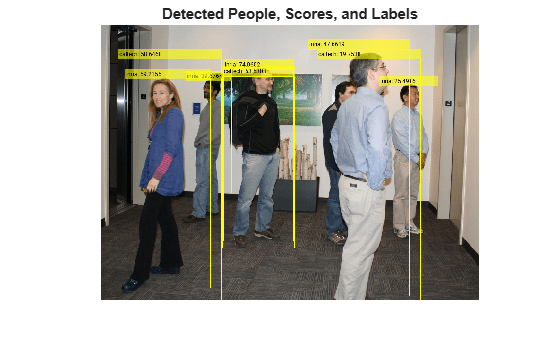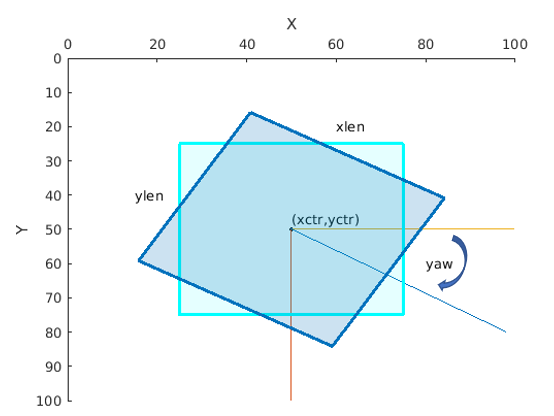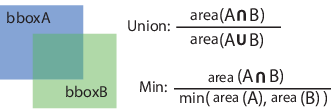# selectStrongestBboxMulticlass

Select strongest multiclass bounding boxes from overlapping clusters

## Syntax

``selectedBboxes = selectStrongestBboxMulticlass(bboxes,scores,labels)``
``````[selectedBboxes,selectedScores,selectedLabels,index] = selectStrongestBboxMulticlass(bboxes,scores,labels)``````
``````[___] = selectStrongestBboxMulticlass(___,Name,Value)``````

## Description

example

````selectedBboxes = selectStrongestBboxMulticlass(bboxes,scores,labels)` returns selected bounding boxes that have high confidence scores. The function uses greedy nonmaximal suppression (NMS) to eliminate overlapping bounding boxes from the `bboxes` input, only if they have the same class label.```
``````[selectedBboxes,selectedScores,selectedLabels,index] = selectStrongestBboxMulticlass(bboxes,scores,labels)``` additionally returns the scores, labels, and index associated with the selected bounding boxes.```
``````[___] = selectStrongestBboxMulticlass(___,Name,Value)``` uses additional options specified by one or more `Name,Value` pair arguments.```

## Examples

collapse all

Create detectors using two different models. These will be used to generate multiclass detection results.

```detectorInria = peopleDetectorACF('inria-100x41'); detectorCaltech = peopleDetectorACF('caltech-50x21');```

Apply the detectors.

```I = imread('visionteam1.jpg'); [bboxesInria,scoresInria] = detect(detectorInria,I,'SelectStrongest',false); [bboxesCaltech,scoresCaltech] = detect(detectorCaltech,I,'SelectStrongest',false);```

Create categorical labels for each the result of each detector.

```labelsInria = repelem("inria",numel(scoresInria),1); labelsInria = categorical(labelsInria,{'inria','caltech'}); labelsCaltech = repelem("caltech",numel(scoresCaltech),1); labelsCaltech = categorical(labelsCaltech,{'inria','caltech'});```

Combine results from all detectors to for multiclass detection results.

```allBBoxes = [bboxesInria;bboxesCaltech]; allScores = [scoresInria;scoresCaltech]; allLabels = [labelsInria;labelsCaltech];```

Run multiclass non-maximal suppression.

```[bboxes,scores,labels] = selectStrongestBboxMulticlass(allBBoxes,allScores,allLabels,... 'RatioType','Min','OverlapThreshold',0.65);```

Annotate detected people.

```annotations = string(labels) + ": " + string(scores); I = insertObjectAnnotation(I,'rectangle',bboxes,cellstr(annotations)); imshow(I) title('Detected People, Scores, and Labels')```## Input Arguments

collapse all

Bounding boxes, specified as an M-by-4 or M-by-5 nonsparse numeric matrix. M is the number of bounding boxes. Each row of the matrix defines a bounding box as either an axis-aligned rectangle or a rotated rectangle. This table describes the format for each bounding box.

Bounding BoxDescription
Axis-aligned rectangle

Defined in pixel coordinates as an M-by-4 numeric matrix with rows of the form [x y w h], where:

• M is the number of axis-aligned rectangles.

• x and `y` specify the upper-left corner of the rectangle.

• w specifies the width of the rectangle, which is its length along the x-axis.

• h specifies the height of the rectangle, which is its length along the y-axis.

Rotated rectangle

Defined in spatial coordinates as an M-by-5 numeric matrix with rows of the form [xctr yctr xlen ylen yaw], where:

• M is the number of rotated rectangles.

• xctr and yctr specify the center of the rectangle.

• xlen specifies the width of the rectangle, which is its length along the x-axis before rotation.

• ylen specifies the height of the rectangle, which is its length along the y-axis before rotation.

• yaw specifies the rotation angle in degrees. The rotation is clockwise-positive around the center of the bounding box.Data Types: `single` | `double` | `int8` | `int16` | `int32` | `uint8` | `uint16` | `uint32`

Confidence scores corresponding to the input bounding boxes, specified as an M-by-1 vector. The `selectStrongestBboxMulticlass` function uses greedy NMS to eliminate overlapping bounding boxes and associate the confidence score with the boxes. A higher score represents a higher confidence in keeping the bounding box. The `scores` input must be real, finite, and nonsparse.

Data Types: `single` | `double` | `int8` | `int16` | `int32` | `uint8` | `uint16` | `uint32`

Labels corresponding to the input bounding boxes, specified as an M-by-1 categorical or numeric vector.

Data Types: `single` | `double` | `int8` | `int16` | `int32` | `uint8` | `uint16` | `uint32` | `categorical`

### Name-Value Arguments

Specify optional comma-separated pairs of `Name,Value` arguments. `Name` is the argument name and `Value` is the corresponding value. `Name` must appear inside quotes. You can specify several name and value pair arguments in any order as `Name1,Value1,...,NameN,ValueN`.

Example: `'RatioType'`,`'Union'` sets the `'RatioType'` property to `'Union'`.

Ratio type, specified as the character vector `'Union'` or `'Min'`.

• Set the ratio type to `'Union'` to compute the ratio as the area of intersection between `bboxA` and `bboxB`, divided by the area of the union of the two.

• Set the ratio type to `'Min'` to compute the ratio as the area of intersection between `bboxA` and `bboxB`, divided by the minimum area of the two bounding boxes.Data Types: `char`

Overlap ratio threshold, specified as the comma-separated pair consisting of '`OverlapThreshold`' and a scalar in the range [0 1]. When the overlap ratio is above the threshold, the function removes bounding boxes around the reference box. Decrease the threshold to reduce the number of selected bounding boxes. However, if you decrease the threshold too much, you might eliminate boxes that represent objects close to each other in the image.

Data Types: `single` | `double`

Maximum number of strongest boxes, specified as the comma-separated pair consisting of `'NumStrongest'` and `inf` or a positive scalar. Use this argument to reduce processing time when you have a priori knowledge about the maximum number of boxes. Set the value to `inf` to select all the strongest, non-overlapping, bounding boxes.

When the `labels` input contains categorical labels, you can also specify a vector that contains the maximum number of strongest boxes for each category in the labels input. The length of the specified vector must equal the number of categories in the label.

## Output Arguments

collapse all

Selected bounding boxes, returned as an M-by-4 or an M-by-5 matrix. The 4-element vectors represent axis-aligned rectangles and the 5-element vectors represent rotated rectangles.

The `selectedBbox` output returns the selected bounding boxes from the `bbox` input that have the highest confidence score. The function uses nonmaximal suppression to eliminate overlapping bounding boxes.

Data Types: `single` | `double` | `int8` | `int16` | `int32` | `uint8` | `uint16` | `uint32`

Scores of selected bounding boxes, returned as an M-by-1 vector. The Mth score in the `selectedScores` output corresponds to the Mth bounding box in the `selectedBboxes` output. The data type of `selectedScores` matches the data type of `scores`.

Labels of selected bounding boxes, returned as an M-by-1 categorical or numeric vector. The Mth label in the `selectedLabels` output corresponds to the Mth bounding box in the `selectedBboxes` output. The data type of `selectedLabels` matches the data type of `labels`.

Index of selected bounding boxes, returned as an M-by-1 vector. The `index` vector contains the indices to the selected boxes in the `bboxes` input.

Data Types: `double`